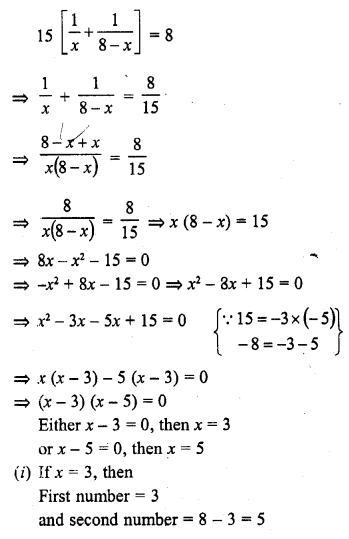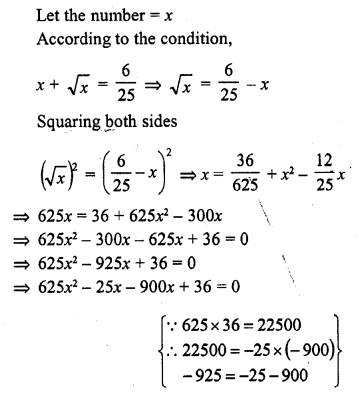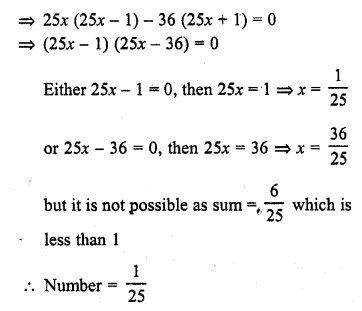• +91 9971497814
• info@interviewmaterial.com

### Related Subjects

Question 1 : Find two consecutive numbers whose squares have the sum 85. (C.B.S.E. 2000)

Let first number = x
Then second number = x + 1
According to the condition
x² + (x + 1)2 = 85
=> x² + x² + 2x + 1 = 85
=> 2x² + 2x + 1 – 85 = 0
=> 2x² + 2x – 84 = 0
=> x² + x – 42 = 0
=> x² + 7x – 6x – 42 = 0
=> x (x + 7) – 6 (x + 7) = 0
=> (x + 7) (x – 6) = 0
Either x + 7 = 0, then x = -7 or x – 6 = 0, then x = 6
(i) If x = -7, then the first number = -7 and second number = -7 + 1 = -6
(ii) If x = 6, then the first number = 6 and second number = 6 + 1 = 7
Hence numbers are -7, -6 or 6, 7

Question 2 : Divide 29 into two parts so that the sum of the squares of the parts is 425.

Total = 29
Let first part = x
Then second part = 29 – x
According to the condition
x² + (29 – x)2 = 425
=> x² + 841 + x² – 58x = 425
=> 2x² – 58x + 841 – 425 = 0
=> 2x² – 58x + 416 = 0
=> x² – 29x + 208 = 0 (Dividing by 2)
=> x² – 13x – 16x + 208 = 0
=> x(x – 13) – 16 (x – 13) = 0
=> (x – 13) (x – 16) = 0
Either x – 13 = 0, then x = 13 or x – 16 = 0, then x = 16
(i) If x = 13, then First part =13 and second part = 29 – 13 = 16
(ii) If x = 16, then First part =16 and second part = 29 – 16 = 13
Parts are 13, 16

Question 3 : Two squares have sides x cm and (x + 4) cm. The sum of their areas is 656 cm2. Find the sides of the squares. (C.B.S.E. 1997)

Side of the first square = x cm
Its area = (side)2 = x² cm2
Side of the second square = (x + 4) cm
Its area = (x + 4)2 cm2
According to the condition,
x² + (x + 4)2 = 656
=> x² + x² + 8x + 16 = 656
=> 2x² + 8x + 16 – 656 = 0
=> 2x² + 8x – 640 = 0
=> x² + 4x – 320 = 0 (Dividing by 2)
=> x² + 20x – 16x – 320 = 0
=> x (x + 20) – 16 (x + 20) = 0
=> (x + 20) (x – 16) = 0
Either x + 20 = 0, then x = -20 Which is not possible being negative
or x – 16 = 0, then x = 16
Side of the first square = 16 cm
and side of the second square = 16 + 4 = 20 cm

Question 4 : The sum of two numbers is 48 and their product is 432. Find the numbers.

Sum of two numbers = 48
Let first number = x
The second number = 48 – x
According to the condition,
x (48 – x) = 432
=> 48x – x² = 432
=> – x² + 48x – 432 = 0
=> x² – 48x + 432 = 0
=> x² – 12x – 36x + 432 = 0
=> x (x – 12) – 36 (x – 12) = 0
=> (x – 12) (x – 36) = 0
Either x – 12 = 0, then x = 12 or x – 36 = 0, then x = 36
(i) If x = 12, then First number = 12 and second number = 48 – 12 = 36
(ii) If x = 36, then First number = 36 and second number = 48 – 36 = 12
Numbers are 12, 36

Question 5 : If an integer is added to its square, the sum is 90. Find the integer with the help of quadratic equation.

Let the given integer be = x
According to the condition
x² + x = 90
=> x² + x – 90 = 0
=> x² + 10x – 9x – 90 = 0
=> x (x + 10) – 9 (x + 10) = 0
=> (x + 10) (x – 9) = 0
Either x + 10 = 0, then x = -10 or x – 9 = 0, then x = 9.
The integer will be -10 or 9

Question 6 : Find the whole number which when decreased by 20 is equal to 69 times the reciprocal of the number.

Let the given whole number = x
Then its reciprocal = 1/x
According to the condition,
x – 20 = 69 x (1/x)
=> x – 20 = 69/x
=> x² – 20x = 69
=> x² – 20x – 69 = 0
=> x² – 23x + 3x – 69 = 0
=> x (x – 23) + 3 (x – 23) = 0
=> (x – 23) (x + 3) = 0
Either x – 23 = 0, then x = 23
or x + 3 = 0, then x = -3, but it is not a whole number
Required whole number = 23

Question 7 : Find two consecutive natural numbers whose product is 20.

Let first natural number = x
Then second number = x + 1
According to the condition,
x (x + 1) = 20
=> x² + x – 20 = 0
=> x² + 5x – 4x – 20 = 0
=> x (x + 5) – 4 (x + 5) = 0
=> (x + 5) (x – 4) = 0
Either x + 5 = 0, then x = -5 which is not a natural number
or x – 4 = 0, then x = 4
First natural number = 4 and second number = 4 + 1=5

Question 8 : The sum of the squares of two consecutive odd positive integers is 394. Find them.

Let first odd number = 2x + 1
Then second odd number = 2x + 3
According to the condition
(2x + 1)2 + (2x + 3)2 = 394
=> 4x² + 4x + 1 + 4x² + 12x + 9 = 394
=> 8x² + 16x + 10 = 394
=> 8x² + 16x + 10 – 394 = 0
=> 8x² + 16x – 384 = 0
=> x² + 2x – 48 = 0 (Dividingby8)
=> x² + 8x – 6x – 48 = 0
=> x(x + 8) – 6(x + 8) = 0
=> (x + 8) (x – 6) = 0
Either x + 8 = 0, then x = 8 but it is not possible as it is negative
or x – 6 = 0, then x = 6
First odd number = 2x + 1 = 2 x 6 + 1 = 13
and second odd number = 13 + 2 = 15

Question 9 : The sum of two numbers is 8 and 15 times the sum of their reciprocals is also 8. Find the numbers.

Sum of two numbers = 8
Let first number = x
Then second number = 8 – x
According to the condition,(ii) If x = 5, then First number = 5 and second number = 8 – 5 = 3
Numbers are 3, 5

Question 10 : The sum of a number and its positive square root is 6/25. Find the number.Todays Deals### RD Chapter 8- Quadratic Equations Ex-8.7 Contributorskrishan

Name:
Email:

# Latest News# 9000 interview questions in different categories